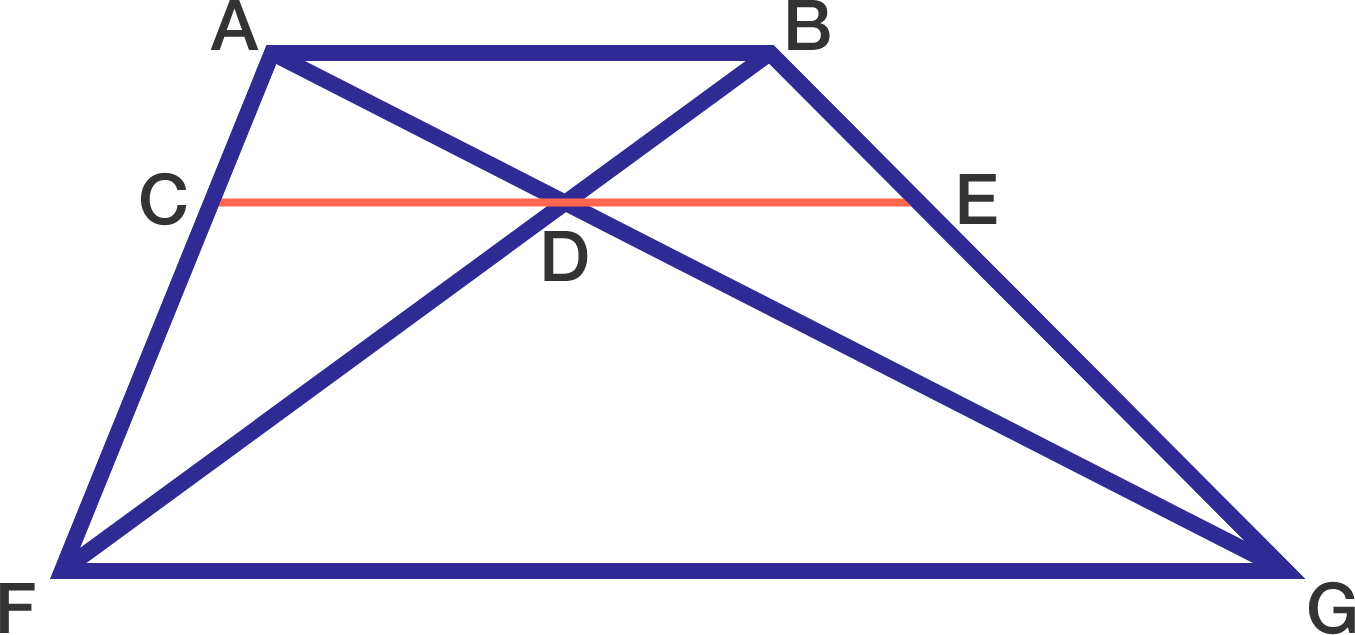# Trapezoid Mystery

Geometry Level 3

In the diagram below, $ABGF$ is a trapezoid and $D$ is the intersection point of its diagonals.

If $CE$ is drawn such that it passes through $D$ and is parallel to $AB,$ is it true that $CD = DE?$×

Problem Loading...

Note Loading...

Set Loading...